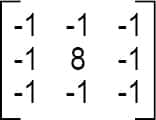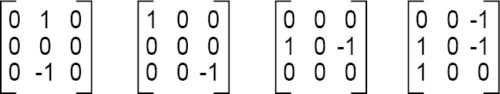# Image Processing Using MATLAB: Spatial Filtering (Part 3 of 4)

Dr Anil Kumar Maini is former director, Laser Science and Technology Centre, a premier laser and optoelectronics R&D laboratory of DRDO of Ministry of Defence

14971

## Linear high-pass filters

Linear HPFs can be implemented using 2D convolution masks with positive and negative coefficients, which correspond to a digital approximation of the Laplacian—a simple, isotropic (rotation-invariant) second-order derivative that is capable of responding to intensity transitions in any direction.

An alternative digital implementation of the Laplacian takes into account all eight neighbours of the reference pixel in the input image and can be implemented by the convolution mask given below:### Directional difference filters

Directional difference filters are similar to the Laplacian high-frequency filter but emphasise edges in a specific direction. There filters are usually called emboss filters. There are four representative masks that can be used to implement the emboss effect:Linear high-pass filters include Prewitt and Sobel operators. These are also referred to as first-order edge-detection methods. High-pass filters are used for edge detection and edge enhancement operations.

The IPT function edge has options for both Prewitt and Sobel operators. Edge detection using Prewitt and Sobel operators can also be achieved by using imfilter command with the corresponding 3×3 masks (which can be created using fspecial command).

Following code extracts edges in the image using Prewitt operator:

>>Prewitt_A=edge(A,’Prewitt’);
>>imshow(Prewitt_A)

Fig. 10 shows the resultant image.

Let us now add Gaussian noise to the Penguins_grey.jpg image. We then process it using the Prewitt edge detector:

>>A_noise=imnoise(A,’gaussian’);
>>Prewitt_A_noise=edge(A_noise,
’Prewitt’);
>>imshow(A_noise)
>>imshow(Prewitt_A_noise)

Fig. 11 shows the image with the added Gaussian noise and Fig. 12 shows the image filtered with Prewitt edge detector.

Following code shows the use of Sobel operator for edge detection:

>>Sobel_A=edge(A,’sobel’);
>>imshow(Sobel_A)

Fig. 13 shows the resultant image.

Let us now add Gaussian noise to the Penguins_grey.jpg image. We then process it using the Sobel edge detector:

>>A_noise=imnoise(A,’gaussian’);
>>Sobel_A=edge(A,’sobel’);
>>imshow(Sobel_A)

Fig. 14 shows the resultant image.

## Non-linear filters

Non-linear filters work at a neighbourhood level, but do not process pixel values using the convolution operator. Instead, these usually apply a ranking (sorting) function to pixel values within the neighbourhood and select a value from the sorted list. For this reason, these are sometimes called rank filters. Examples of non-linear filters include median filter and max and min filters.

Median filter is a popular non-linear filter used in image processing. It works by sorting the pixel values within a neighbourhood, finding the median value, and replacing the original pixel value with the median of that neighbourhood. Median filter works much better than an averaging filter with comparable neighbourhood size in reducing salt and pepper noise. Because of its popularity, median filter has its own function (medfilt2) provided by the IPT.

Following code shows the use of median filter to remove salt and pepper noise from the image Penguins_grey.jpg:

Noise_snp=imnoise(A,’salt& pepper’);
imshow(Noise_snp)
Filtered_snp=medfilt2(Noise_snp,[3 3]);
imshow(Filtered_snp)

Figs 15 and 16 show noisy and cleaned images, respectively. Fig. 17 shows another noisy image and Fig. 18 shows this image cleaned using median filter.

## Sliding window operations

Sliding window neighbourhood operations are implemented in the IPT using one of these two functions: nlfilter or colfilt. Both functions accept a user-defined function as a parameter. Such function can perform linear as well as non-linear operations on the pixels within a window. For rank filters, the IPT function ordfilt2 makes it very easy to create min, max and median filters.

The syntax for nlfilter has three arguments: the image matrix, the size of the filter and the function to be applied. The following line defines a filter that takes the maximum value in a 3×3 neighbourhood:

cmax = nlfilter(c,[3,3],‘max(x(:))’);

A filter that takes minimum value in a 3×3 neighbourhood is defined by the following command:

cmin = nlfilter(c,[3,3],‘min(x(:))’);

## Region of interest processing

Filtering operations are sometimes performed only in a small part of an image, referred to as the region of interest (ROI). ROI is specified by defining a mask that limits the portion of the image in which the operation will take place.

Image masking is the process of extracting a sub-image from a larger image for further processing. ROI processing can be implemented in MATLAB using a combination of two functions: roipoly (for image masking) and roifilt2 (for actual processing of the selected ROI).

To be continued…